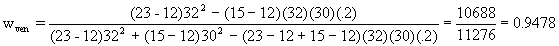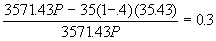Fin 652 INVESTMENT ANALYSIS

Fall 1997

Midterm

• The quiz is closed book.
• Time allowed is 1 hour and 30 minutes.
• Explain all your steps; correct answers without explanations may not be given any credit at all.
• Show all your computations and formulae. Make all your assumptions explicit. If your approach is correct, you will get some credit, even if your arithmetic answer is wrong. So concentrate on getting your logic right.
• If you answer a question, I have the discretion to award you some points, even if you are completely wrong. If you don't attempt the question at all, I can give you no points! So attempt every question.

1. (based on Problem 16 of chapter 8) During a particular time period, the riskfree rate was 8%, while the average return on the market portfolio was 20%, with a standard deviation of returns of 25%. Investor A's portfolio had a beta of 1.5, with an average return of 16%, while investor B's portfolio had a beta of 2.0, with an average return of 23%. Which of the two investors did a better job of picking stocks? Assuming that the investors picked portfolios with the betas that they wanted, what could they have done to improve their expected return? (Answer using the information that you have available to you in the question. I don't want general advice.)

2. (Similar to prob. 6, p. 223, chapter 7)You have available to you, two mutual funds, whose returns have a correlation of 0.2. Here is some information on their return probability distributions:

 Expected Return Standard Deviation Ventura 23% 30% Marco Polo 15% 32%

In addition, you can also invest in a riskfree 1-year T-bill yielding 12%

1. If you have a risk aversion coefficient of 4, and you have a total of \$20,000 to invest, how much should you invest in each of the three investment vehicles?
2. What is the standard deviation of your optimal portfolio?
3. If you wanted an expected return of 50% with as low a portfolio variance as possible, what would you invest in each of the three investment vehicles? Assume for the sake of this question that you cannot borrow at the riskfree rate.

3. Bret Barakett has a margin account and deposits \$50,000. Assuming the prevailing initial margin requirement is 40%, commissions are ignored, and Reebok is selling at \$35 per share:

1. How many shares can Brett purchase using the maximum allowable margin?
2. What is Brett's profit/loss if the price of Reebok stock falls to \$25?
3. If the maintenance margin is 30%, to what price can Reebok fall before Brett will receive a margin call?
4. How much additional money must Brett deposit if the stock price drops to \$5 below the level computed in c) above, and he is required to bring up the actual margin on his account to the initial margin?

Solutions:

1.

E(RA)=.08+1.5(.20-.08) = 0.26; Jensen's alpha = .26-.16 = -.10

E(RB)=.08+2(.20-.02) = 0.32; Jensen's alpha = .32-.23 = -.09

According to Jensen's measure, B is better.

Both of them could have done better by borrowing at 8% and investing in the market portfolio. Thus, A should have borrowed 50% of his original investment and invested all of it in the market portfolio, while B should have borrowed an amount equal to his original investment and invested it in the market portfolio.

2. a., b.The tangent portfolio is given bywmp = 0.0521.

The variance of returns of this portfolio is .94782(30)2+.05212322+2(.0521)(.9478)(30)(32)(.2) = 830.2389, and a standard deviation of returns of 28.81. The expected return is 23(.9478) + (15)(.0521) = 22.5809.

The optimal amount to invest in this portfolio is (22.58-12)/(.01)(4)(830.2389) = 0.3186. Hence, we find that the final investment proportions are .3186(.9478) = .3020 in Ventura, .3186(.0521)=.0166 in Marco Polo and .6814 or the rest in the riskfree asset.

c. To get an expected return of 50% without shortselling the riskfree asset, the only way is to shortsell the Marco Polo fund. Solve 0.5 = .15wMP + .23(1- wMP) to get wMP = -3.375 and wv = 4.375

3. a. Brett can buy 50000/(.4)(35) or 3571.43 shares.

b.If the price falls to \$25, the loss is (35-25)(3571.43) or 35714.30.

c. The price can fall up P, where P is given by, or P = \$30.

d. If the price falls to \$25, he needs to have 0.4(25)(3571.43) or \$35714.30; he originally had \$50,000, of which he lost 35714.30. Hence he now needs an additional 35714.30 - (50000-35714.30) = \$21428.

Final Exam

• The exam is closed book.
• Explain all your steps; correct answers without explanations may not be given any credit at all.
• Show all your computations and formulae. Make all your assumptions explicit. If your approach is correct, you will get some credit, even if your arithmetic answer is wrong. So concentrate on getting your logic right.
• Write legibly.
• If an answer contains two contradictory statements, you may get no credit for the answer, even if one of the statements is correct.
• If you answer a question, I have the discretion to award you some points, even if you are completely wrong. If you don't attempt the question at all, I can give you no points! So attempt every question.

1. The following bonds are available at the present time (making annual coupon payments)

 Bond Maturity Coupon Price A 1 0% 909.09091 B 2 5% 913.22314 C 3 10% 1000 D 4 0% 683.01346
1. If the expectations theory of the yield curve is correct, what is the market expectation of the price that Bond C will sell for next year?
2. If you believe that the liquidity premium is 1% per annum on all bonds with a maturity greater than one year, what is your prediction for the spot rate on one year bonds next year?
3. If you believe that the liquidity premium is 1% per annum on all bonds, and likely to remain at 1% for the foreseeable future, what is your prediction of the spot rate on two year bonds next year?

2. You have the following obligations and expected cash inflows for the next 5 years:

 Time In 1 year In 2 years In 3 years In 4 years Obligation \$0 \$10,000 \$20,000 \$900 Cash Inflow \$0 \$5,000 \$12,000 \$0
1. What is the duration of my net obligations? You may use term structure information from Question 1.
2. You can use bonds B and C above to immunize your portfolio. How much should you invest in each of them? (Answer in dollars).
3. If you could use bonds A and D as well, would you use them? Why or why not? (Bonus)

3. Consider the following information regarding the performance of a fund manager in a recent month. The table represents the actual return of each sector of the manager’s portfolio in Column 1, the fraction of the portfolio allocated to each sector in Column 2, the benchmark or neutral sector allocations in Column 3, and the returns of sector indices in Column 4.

 Actual Return Actual Weight Benchmark Weight Index Return Equity 2% .70 .60 2.5% Bonds 1% .20 .30 1.2% Cash 0.5% .10 .10 0.4%
1. What was the manager’s return during the month? What was her overperformance or underperformance?
2. What was the contribution of security selection to relative performance?
3. What would be the best way to use this manager’s skills?

Solution:

1. It is easy to determine that the yield on all bonds is 10%; hence the term structure is flat at 10%.

1. This being the case, bond C next year will be a 2 year bond with a coupon of 10% and will sell at a price equal to the present value of the coupons and face value, discounted at 10%. However, since the coupons are also 10%, the bond will still sell at par, i.e. \$1000.
2. Since the expected future spot rate equals the forward rate less the liquidity premium, the expected future spot rate is 10% - 1% or 9%.
3. Since the liquidity premium is 1% at all maturities, our prediction for all future spot rates is 9%. The return on a one year zero-coupon bond two years from is , therefore, predicted to be 9% (since that bond will have no liquidity premium). The one-year return on a two year zero-coupon bond, one year from now should be 10%, since this return should be equal to the return on a one-year zero-coupon bond at that time (9%) plus the liquidity premium of 1%. Hence the total return over the two year period on a two-year zero-coupon bond to be issued next year can be estimated at (1.10)(1.09) - 1 or 19.9%; the yield on that bond will, therefore, be the square root of 1.199 or 9.499%.

2. a. The term structure is known to be flat at 10%. Using this information, the duration of the net obligations of the individual works out to 2.68. The present value of the net obligations equals \$10,825.80.

 Year 1 2 3 4 Outflow (10,000.00) \$(20,000.00) \$(1,000.00) Inflow \$ 5,000.00 \$ 12,000.00 Net flow \$0 (\$5,000) (\$8,000) (\$1,000) PV 0 -4132.2314 -6010.51841 -683.01346 [PV/Sum(PV)]xYear 0 0.76340694 1.66561514 0.2523659

The durations of the bonds can be worked out more easily; for bonds A and D, the duration equals the maturity, for bond C, which is selling at par, rule 8 can be used; for bond B, rule 7 can be used.

 Bond Maturity coupon Price Duration A 1 0% 909.090909 1 B 2 5% 913.22314 1.95 C 3 10% 1000 2.74 D 4 0% 683.013455 4

b. We need to create portfolios with durations equal to 2.68. Since the yields of all the bonds are the same, the duration of any portfolio is equal to the value-weighted average of the individual bonds. Hence, if we invest y percent in bond B and (1-y) percent in bond C, where 1.95y + 2.74(1-y) = 2.68, we’d be able to immunize the net obligations. Solving, we get y = 7.59%. That is, we would invest 7.59% of 10825.80, or \$821.68 in Bond B and \$10,004.12 in Bond C.

c. If it were possible to use bonds A and D, I would use them for two reasons: one, I would get better convexity properties, because the cashflows from the bond portfolio would be more dispersed. Secondly, it would be possible to shield against interest rate risk completely by constructing a portfolio using bonds A, B, C, and D so that the net flows in each period from the portfolio equal the net obligations in that period.

3.

1. The manager’s return is given by 0.7(2) + .2(1) + .1(0.5) = 1.65%
2. Security selection is computed as 1.65% - [.7(2.5) + .2(1.2) + .1(0.4)] = -0.38%
3. This implies that market timing is 0.13%, since total underperformance is only 0.25%. Hence I would use the manager in asset allocation, rather than in security selection.